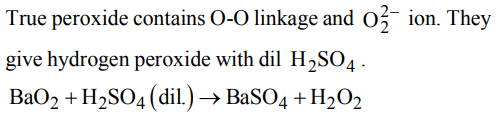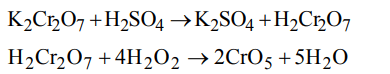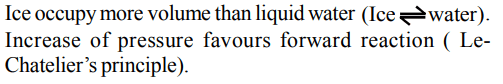## Hydrogen Questions and Answers Part-4

1. The critical temperature of water is higher than that of $O_{2}$ because $H_{2}O$  molecule has
a) fewer electrons than oxygen
b) two covalent bonds
c) v-shape
d) dipole moment

Explanation: Critical temperature of water is more than O2 due to its dipole moment (Dipole moment of water = 1.84 D; Dipole moment of O2 = zero).

2. Hydrogen is not obtained when Zn reacts with
a) cold water
b) dil $H_{2}SO_{4}$
c) dil. HCl
d) 20% NaOH

Explanation: Only elements having reduction potential less than - 0.41V liberate hydrogen with cold water.

3. True peroxide is
a) $BaO_{2}$
b) $MnO_{2}$
c) $PbO_{2}$
d) $NO_{2}$

Explanation:4. An inorganic compound gives off O2 when heated, turns an acidic solution of KI violet and reduces acidified $KMnO_{2}$  . The compound is
a) $SO_{3}$
b) $KNO_{3}$
c) $H_{2}O_{2}$
d) All of these

Explanation:5. Acidified solution of chromic acid on treatment with $H_{2}O_{2}$   yields
a) $CrO_{3}+H_{2}O+O_{2}$
b) $Cr_{2}O_{2}+H_{2}O+O_{2}$
c) $CrO_{5}+H_{2}O+K_{2}SO_{4}$
d) $H_{2}Cr_{2}O_{7}+H_{2}O+O_{2}$

Explanation:6. The m.pt. of most of the solid substances increase with an increase of pressure. However ice melts at a temperature lower than its usual melting point when pressure is increased. This is because
a) ice is less denser than $H_{2}O$
b) pressure generates heat
c) the chemical bonds break under pressure
d) ice is not a true solid

Explanation:7. Acidified K2Cr2O7 on oxidation by $H_{2}O_{2}$ gives
a) Blue solution
b) $CrO_{5}$
c) Chromium peroxide
d) All of these

Explanation:8.Which reaction shows oxidising nature of $H_{2}O_{2}$
a) $H_{2}O_{2}+2KI\rightarrow 2KOH+I_{2}$
b) $Cl_{2}+H_{2}O_{2}\rightarrow2HCl+O_{2}$
c) $H_{2}O_{2}+Ag_{2}O\rightarrow2Ag+H_{2}O+O_{2}$
d) $NaClO+H_{2}O_{2}\rightarrow NaCl+H_{2}O+O_{2}$

Explanation:9. The volume strength of 0.5 N $H_{2}O_{2}$ solution is
a) 4.8
b) 8.4
c) 3.0
d) 8.0